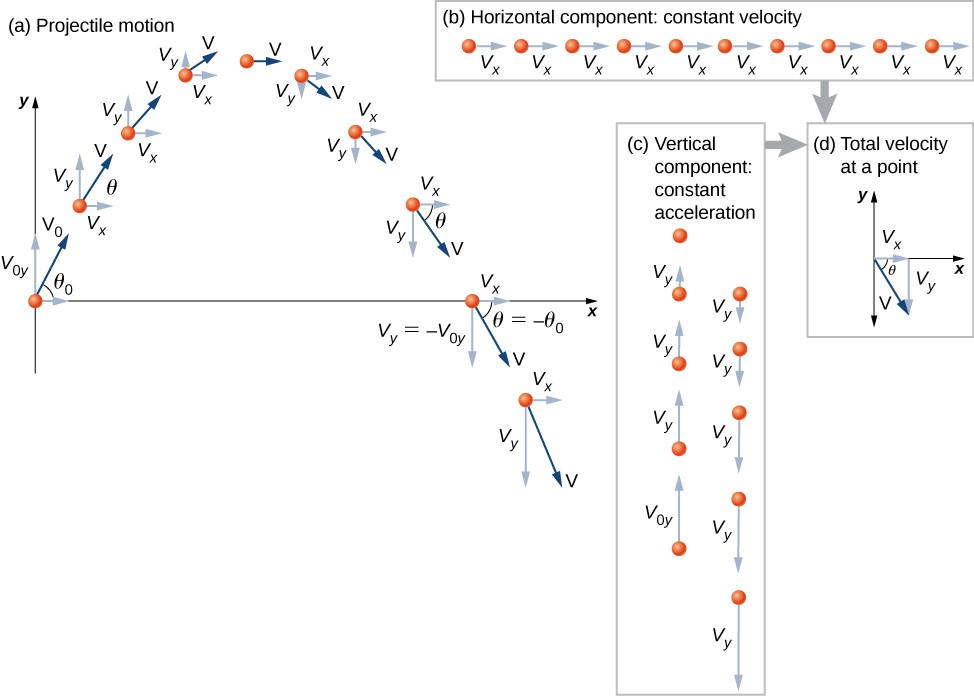## Projectile Motion

• Projectile motion is the motion of an object thrown or projected into the air, subject to only the acceleration of gravity. The object is called a projectile, and its path is called its trajectory.

• Note that motions along perpendicular axes are independent and thus can be analyzed separately. The key to analyzing a two-dimensional projectile motion is to break it into two motions, one along the horizontal axis and the other along the vertical axis.

### Analyzing Projectile Motion

• Step 1 Resolve the motion into horizontal and vertical components along the $x$- and $y$-axes. The magnitude of the components of displacement $s$ along these axes are $x$ and $y$. The magnitudes of the components of the velocity $v$ are $v_x=v\cos \theta$ and $v_y=v\sin \theta$, where $v$ is the magnitude of the velocity and $\theta$ is the angle with the positive $x$-axis. Note that initial values are denoted with a subscript $0$. For example, the magnitude of the initial velocity is $v_0$ and its $x$- and $y$- components are:

$\begin{matrix} v_{0x} = v_0\cos\theta \\ v_{0y} = v_0\sin\theta \end{matrix}$• Step 2 Treat the motion as two independent one-dimensional motions, one horizontal and the other vertical. The kinematic equations for horizontal and vertical motion take the following forms:

Horizontal Motion  $$\boxed{x = x_0 + v_xt}$$ (1)
 $$\boxed{v_x = v_{0x}= \text{constant} }$$ (2)

Vertical Motion (assume $+$ upward and $g = 9.81~\text{m/s}^2$)

 $$\boxed{a_y = -g = -9.81~\text{m/s}^2}$$ (3)
 $$\boxed{y = y_0 + v_{0y}t - \frac{1}{2}gt^2 }$$ (4)
 $$\boxed{v_y = v_{0y} - gt}$$ (5)
 $$\boxed{v_y^2 = v_{0y}^2 - 2g(y-y_0)}$$ (6)
• Step 3 Solve for the unknowns in the two separate motions—one horizontal and one vertical. Note that the only common variable between the motions is time $t$.

• Step 4 Recombine quantities in the $x$- and $y$-directions to find the total displacement $\bm{s}$ and velocity $\bm{v}$. Solve for the magnitude and direction of $\bm{s}$ and $\bm{v}$ using:

 $$\boxed{s = \sqrt{x^2 + y^2}}$$ (7)
 $$\boxed{\theta = \tan^{-1}\left(\frac{y}{x}\right) }$$ (8)
 $$\boxed{v = \sqrt{v_x^2 + v_y^2}}$$ (9)
 $$\boxed{\theta_v = \tan^{-1}\left(\frac{v_y}{v_x}\right) }$$ (10)

### Examples

EXAMPLE 1: Projectile motion.

A projectile is launched at ground level with an initial speed of $50.0~$m/s at an angle of $30.0^\circ$ above the horizontal. It strikes a target above the ground $3.00~$s later. What are the $x$ and $y$ distances from where the projectile was launched to where it lands?

Solution (click to expand)
130m horizontally
30.9m vertically

EXAMPLE 2: Projectile motion.

A ball is kicked with an initial velocity of $16~$m/s in the horizontal direction and $12~$m/s in the vertical direction.

(a) At what speed does the ball hit the ground?
(b) For how long does the ball remain in the air?
(c) What maximum height is attained by the ball?

Solution (click to expand)
(a) 20m/s
(b) 2.45s
(c) 7.35m

EXAMPLE 3: Projectile motion.

A ball is thrown horizontally from the top of a $60.0$-m building and lands $100.0~$m from the base of the building. Ignore air resistance.

(a) How long is the ball in the air?
(b) What must have been the initial horizontal component of the velocity?
(c) What is the vertical component of the velocity just before the ball hits the ground?
(d) What is the speed and direction of the ball just before it hits the ground?

Solution (click to expand)
(a) 3.50s
(b) 28.6m/s
(c) 44.7m/s
(d) 50.2$^\circ$ below $x$-axis